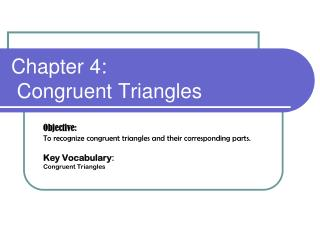DownloadDownload PresentationChapter 4: Congruent Triangles

# Chapter 4: Congruent Triangles

Download Presentation## Chapter 4: Congruent Triangles

- - - - - - - - - - - - - - - - - - - - - - - - - - - E N D - - - - - - - - - - - - - - - - - - - - - - - - - - -
##### Presentation Transcript

1. Chapter 4: Congruent Triangles Objective: To recognize congruent triangles and their corresponding parts. Key Vocabulary: Congruent Triangles

2. Are the triangles congruent?

3. 4.2 Triangle CongruenceSSS and SAS To Prove two triangles congruent using SSS and SAS postulate

4. 1. 2. 3. 4.

5. Triangle Congruence By ASA and AAS Objective: To prove two triangles congruent using ASA Postulate and the AAS Theorem

6. Congruent Triangles

7. Which two triangles are congruent? Explain.

8. 4.4 Using Corresponding Parts of Congruent Triangles

9. 4.4 Using Corresponding Parts of Congruent Triangles

10. 4.4 Using Corresponding Parts of Congruent Triangles

11. 4.4 Using Corresponding Parts of Congruent Triangles

12. 4.5 Isosceles and Equilateral Triangles Objective: To use and apply properties of isosceles and equilateral triangles

13. 4.5 Isosceles and Equilateral Triangles

14. 4.6 Congruence in Right Triangles Objective To prove right triangles congruent using the Hypotenuse Leg Theorem